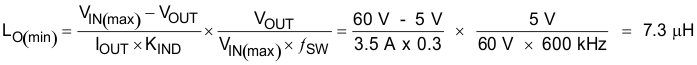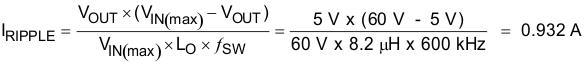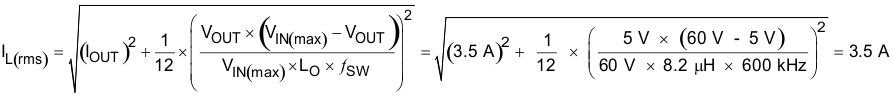JAJSBY0G August   2012  – June 2018

PRODUCTION DATA.

1. 特長
2. アプリケーション
3. 概要
1.     Device Images
4. 改訂履歴
5. Pin Configuration and Functions
6. Specifications
7. Detailed Description
1. 7.1 Overview
2. 7.2 Functional Block Diagram
3. 7.3 Feature Description
4. 7.4 Device Functional Modes
8. Application and Implementation
1. 8.1 Application Information
2. 8.2 Typical Application
1. 8.2.1 Design Requirements
2. 8.2.2 Detailed Design Procedure
3. 8.2.3 Application Curves
9. Power Supply Recommendations
10. 10Layout
1. 10.1 Layout Guidelines
2. 10.2 Layout Example
11. 11デバイスおよびドキュメントのサポート
12. 12メカニカル、パッケージ、および注文情報

• DDA|8
• DDA|8

#### 8.2.2.3 Output Inductor Selection (LO)

To calculate the minimum value of the output inductor, use Equation 28.

KIND is a ratio that represents the amount of inductor ripple current relative to the maximum output current. The inductor ripple current is filtered by the output capacitor. Therefore, choosing high inductor ripple currents impacts the selection of the output capacitor since the output capacitor must have a ripple current rating equal to or greater than the inductor ripple current. In general, the inductor ripple value is at the discretion of the designer, however, the following guidelines may be used.

For designs using low ESR output capacitors such as ceramics, a value as high as KIND = 0.3 may be desirable. When using higher ESR output capacitors, KIND = 0.2 yields better results. Since the inductor ripple current is part of the current mode PWM control system, the inductor ripple current should always be greater than 150 mA for stable PWM operation. In a wide input voltage regulator, it is best to choose relatively large inductor ripple current. This provides sufficienct ripple current with the input voltage at the minimum.

For this design example, KIND = 0.3 and the minimum inductor value is calculated to be 7.3 μH. The nearest standard value is 8.2 μH. It is important that the RMS current and saturation current ratings of the inductor not be exceeded. The RMS and peak inductor current can be found from Equation 30 and Equation 31. For this design, the RMS inductor current is 3.5 A and the peak inductor current is 3.97 A. The chosen inductor is a WE 7447797820, which has a saturation current rating of 5.8 A and an RMS current rating of 5.05 A.

As the equation set demonstrates, lower ripple currents will reduce the output voltage ripple of the regulator but will require a larger value of inductance. Selecting higher ripple currents will increase the output voltage ripple of the regulator but allow for a lower inductance value.

The current flowing through the inductor is the inductor ripple current plus the output current. During power up, faults or transient load conditions, the inductor current can increase above the peak inductor current level calculated above. In transient conditions, the inductor current can increase up to the switch current limit of the device. For this reason, the most conservative design approach is to choose an inductor with a saturation current rating equal to or greater than the switch current limit of the TPS54360 which is nominally 5.5 A.

Equation 28.spacer

Equation 29.spacer

Equation 30.spacer

Equation 31.